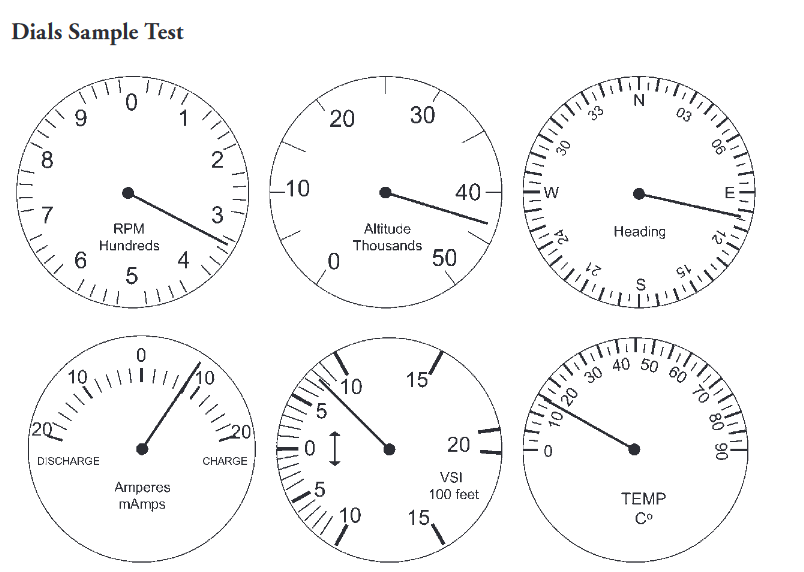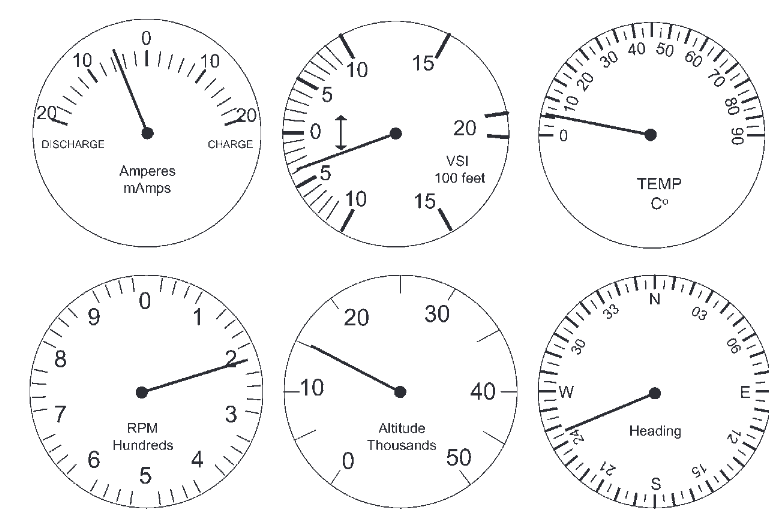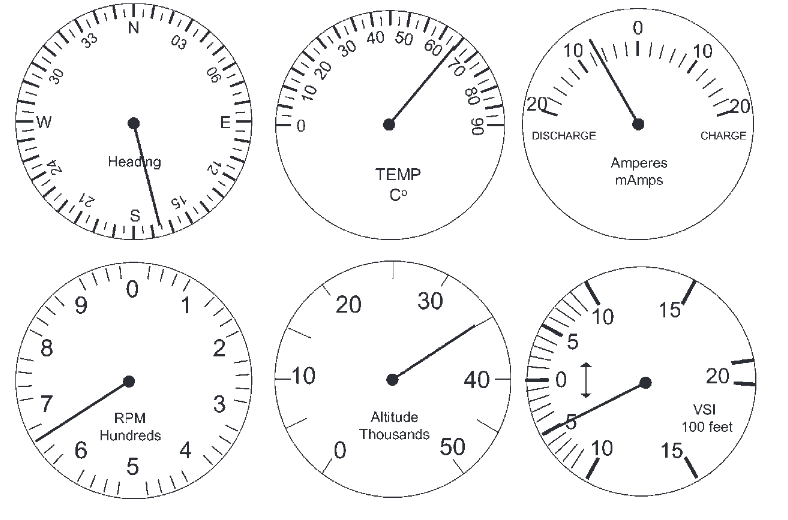# ATSA Dial Reading Practice Test 2023: Air Traffic Controller Aptitude Test

ATSA Dial Reading Practice Test 2023: Air Traffic Controller Aptitude Test: Try our free Air Traffic Selection and Training Aptitude Test (AT-SAT) Dial Reading review question answers. It will help your ATSA Test Prep better. “Air traffic controllers must be able to perceive visual information quickly and accurately and perform simple processing tasks like comparisons. The Dial Reading test assesses the ability to read dials quickly and accurately.”

The Dial Reading test measures the subject’s ability to quickly identify and accurately read certain dials on an instrument panel. Subjects are asked to choose from one of five answers for each question about a given display.

The test consists of 20 questions. Individuals pace themselves against the display of time remaining in the subtest. They are advised to skip difficult items and return them to them at the end of the test. Each panel consists of seven dials in two rows. Each of the seven dials contains unique flight information, e.g., airspeed, fuel, and temperature.

## ATSA Dial Reading Practice TestQ1. What is the ampere reading? _____ milliamps

• A. 8 charge
• B. 8 discharge
• C. 10 charge
• D. 10 discharge

Q2. What is the Vertical Speed Indicator (VSI) reading?

• A. 8000 climb
• B. 800 descend
• C. 8000 descend
• D. 800 climb

Q3. What is the temperature?

• A. 15 F
• B. -15 F
• C. 15 C
• D. -15 C

• A. 185
• B. 105
• C. 115
• D. 095

Q5. What is the altitude?

• A. 40000
• B. 4400
• C. 44000
• D. 50000

Q6. What are the revolutions per minute (RPM)?

• A. 325
• B. 300
• C. 900
• D. 380Q7. What is the Vertical Speed Indicator (VSI) reading?

• A. 4,000 descend
• B. 600 descend
• C. 400 descend
• D. 4,000 climb

• A. 185
• B. 245
• C. 250
• D. 360

Q9. What is the temperature?

• A. 10 F
• B. 10 C
• C. 5 F
• D. 5 C

Q10. What is the altitude?

• A. 20,000
• B. 15,000
• C. 1500
• D. 12,500

Q11. What is the ampere reading? _____ milliamps

• A. 5 charge
• B. 5 discharge
• C. 12 charge
• D. 12 discharge

Q12. What are the revolutions per minute (RPM)?

• A. 520
• B. 250
• C. 180
• D. 200• A. 170
• B. 165
• C. 160
• D. 345

Q14. What is the temperature?

• A. 65 C
• B. 75 C
• C. 65 F
• D. 75 F

Q15. What are the revolutions per minute (RPM)?

• A. 700
• B. 670
• C. 660
• D. 6600

Q16. What is the altitude?

• A. 30,000
• B. 35,000
• C. 3,500
• D. 40,000

Q17. What is the Vertical Speed Indicator (VSI) reading?

• A. 500 descend
• B. 5,000 descend
• C. 5,000 climb
• D. 500 climb